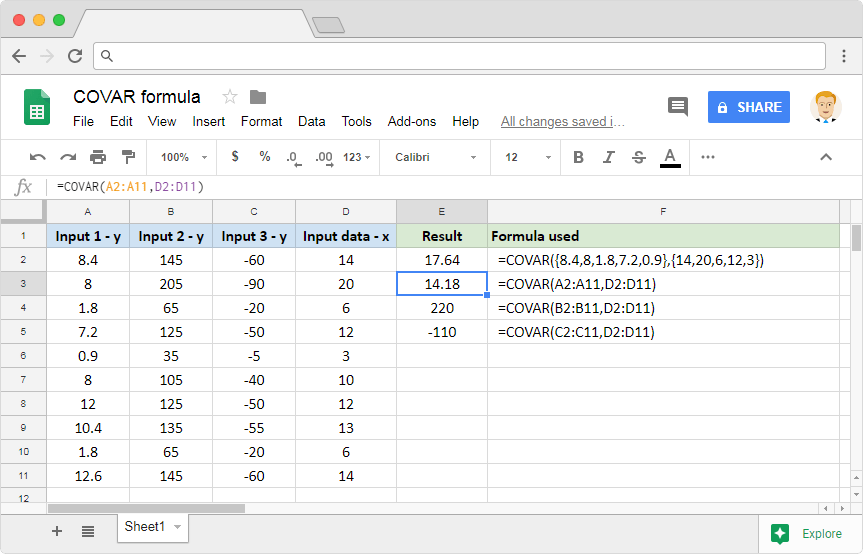# How to use the COVAR formula in Google SheetsAnalyzing the data leads us to multiple interpretations as to what the underlying nature is. And we have many important statistical tools and metrics that help us with this exercise. One such important metric that mathematicians have at their disposal is the covariance. It measures the joint variability of two random variables. Given two data sets, we can calculate this using the readily available COVARformula in Google Sheets.

If the covariance is positive, it indicates that the variables tend to change together in the same direction. Whereas, the negative covariance indicates that they tend to change together in the opposite direction (i.e. increase in one leads to decrease in the other). If you are interested in understanding how we mathematically calculate the covariance, here’s the link to it.

### Syntax

COVAR(data_y, data_x)

• data_y – is the range of values or a reference to the range of cells that consists of the dependent data.
• data_x – is the range of values or a reference to the range of cells that consists of the independent data.

### Usage: COVAR formula in Google Sheets

Now let us go ahead and dive right into the practical application of this formula. Because examples always help us to reinforce our understanding. Please go through the following snapshot taken off the Google Sheets application.The parameters accept the input values in two different ways. We can either choose to input the direct numeric datasets using curly braces that indicate ranges (first example). Or we can simply use range references (final three example).

You’ll notice that we have used three different sets of y data against a single x dataset. There is no particular relation between the datasets in columns A and D. But, observe the data sets in columns B and C. And compare them with that of column D. You’ll see they have positive and negative linear relationships respectively. The sign of the covariance shows the tendency in the linear relationship between the variables. This was evident from the third and fourth examples.

However, it is not straightforward to interpret the magnitude of the covariance. That is because it is not normalized and hence depends on the magnitudes of the variables. If we need to gauge the strength of the linear relationship though, we can use the CORREL formula.